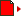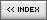A* Pathfinding
[ August 13, 2003 ] by Zeh Fernando
In this example Zeh shows a complete implementation of the A* pathfinding algorithm.

Click on the map to calculate the path starting from the soldier position

This function finds the path between two given points using an A* algorithm. It's usually needed on games that need pathfinding/navigating AI.

It uses a bidimensional map (the MAP), a START point (defined as an Y and X value, in this order to maintain standard with the way the map is built), and an END point (Y and X values).
Each MAP cell have a value of 0 (when it means a wall, or a cell it can't be visited on the route) or a value of 1 or greater. In this case, this value means the COST to navigate through that point. On an standard map, for example, a value of 1 would be used for a road, a value of 2 for normal terrain, and a value of 3 for mountains.
You can also modify the "CONSTANT variables" inside the function. For instance, you can set ALLOW_DIAGONAL to to TRUE to allow diagonal movements or to FALSE to disallow it.

AN EXAMPLE OF USAGE

```// Creates a map

map = [[2,2,2,2,2,2,2,2,2,2,1,2,2,2,2],       [2,2,2,2,2,2,2,2,2,2,1,2,2,2,2],       [2,2,2,2,2,2,2,2,2,2,1,2,2,2,2],       [2,2,2,2,2,2,2,2,2,2,1,2,2,2,2],       [2,2,2,2,2,2,2,2,2,2,1,2,2,2,2],       [2,2,2,2,2,2,2,2,2,2,1,2,2,2,2],       [2,2,0,0,0,0,0,0,0,2,1,2,2,2,2],       [2,2,2,2,2,2,2,2,2,2,1,2,2,2,2],       [2,2,2,2,2,2,2,2,2,2,1,2,2,2,2],       [2,2,2,2,2,2,2,2,2,2,1,2,2,2,2],       [2,2,2,2,2,2,2,2,2,2,1,2,2,2,2],       [2,2,2,2,2,2,2,2,2,2,1,2,2,2,2]];

// Finds the path from point 2,2 to point 9,9

fpath = findPath (map, 2, 2, 9, 9);

// Draw results

for (i=0; i<map.length; i++) {   for (j=0; j<map.length; j++) {      switch (map[i][j]) {      case 0:         _root.beginFill (0x333366);         break;      case 2:         _root.beginFill (0xdddddd);         break;      case 1:         _root.beginFill (0xeeeeee);         break;      }      _root.moveTo (j*20, i*20);      _root.lineTo ((j+1)*20,i*20);      _root.lineTo ((j+1)*20,(i+1)*20);      _root.lineTo (j*20,(i+1)*20);      _root.lineTo (j*20,i*20);      _root.endFill();   }}
for (i=0; i<fpath.length; i++) {   x = fpath[i];   y = fpath[i];   _root.beginFill (0x993333, 70);   _root.moveTo (x*20, y*20);   _root.lineTo ((x+1)*20,y*20);   _root.lineTo ((x+1)*20,(y+1)*20);   _root.lineTo (x*20,(y+1)*20);   _root.lineTo (x*20,y*20);   _root.endFill();}```

THE SOURCE CODE

Note: some lines (where you see "»") in the following script have been broken for page layout reasons.

```/*================================================================================ PATHFINDING FUNCTION=------------------------------------------------------------------------------= Version: 1.0.2= Last changes: jul 15 (1.0.0) - first version= Last changes: aug 09 (1.0.1) - several speed improvements, thanks tonypa= Last changes: aug 10 (1.0.2) - even more speed improvements by tonypa (again)=------------------------------------------------------------------------------= This function finds the path between two points (the START and the END point)= on a given map, taken into account walls/obstacles and terrain costs.== It uses an A* ("a star") algorithm with heuristic approximation for a faster= result. Much of this function is basic on theory learnt from this language= independent tutorial:= http://www.policyalmanac.org/games/aStarTutorial.htm=------------------------------------------------------------------------------= How to use:== my_path_array = findPath (map, start_y, start_x, end_y, end_x)== Parameters:=  map = bidimensional array with rows and columns. Each cell contains a value=        of 0 (wall) or 1+ (terrain - the higher, the more expensive to ride)===============================================================================*/_global.findPath = function(map, startY, startX, endY, endX) {  // Finds the way given a certain path  // Constants/configuration - change here as needed! -------------------------  var HV_COST = 10; // "Movement cost" for horizontal/vertical moves  var D_COST = 14; // "Movement cost" for diagonal moves  var ALLOW_DIAGONAL = true; // If diagonal movements are allowed at all  var ALLOW_DIAGONAL_CORNERING = true; // Not implemented. Always true  // Complimentary functions ==================================================      isOpen = function (y, x) {    // Return TRUE if the point is on the open list, false if otherwise    return mapStatus[y][x].open;  };  isClosed = function (y, x) {    // Return TRUE if the point is on the closed list, false if otherwise    return mapStatus[y][x].closed;  };  nearerSquare = function() {    // Returns the square with a lower movementCost + heuristic distance    // from the open list    var minimum = 999999;    var indexFound = 0;    var thisF = undefined;    var thisSquare = undefined;    var i = openList.length;    // Finds lowest    while (i-->0) {      thisSquare = mapStatus[openList[i]][openList[i]];      thisF = thisSquare.heuristic + thisSquare.movementCost;      if (thisF <= minimum) {        minimum = thisF;        indexFound = i;      }    }    // Returns lowest    return indexFound;  };  closeSquare = function(y, x) {    // Drop from the open list    var len = openList.length;    for (var i=0; i < len; i++) {      if (openList[i] == y) {        if (openList[i] == x) {          openList.splice(i, 1);          break;        }      }    }    // Closes an open square    mapStatus[y][x].open = false;    mapStatus[y][x].closed = true;  };  openSquare = function(y, x, parent, movementCost, heuristic, replacing) {    // Opens a square    if (!replacing) {      openList.push([y,x]);      mapStatus[y][x] = {heuristic:heuristic, open:true, closed:false};    }    mapStatus[y][x].parent = parent;    mapStatus[y][x].movementCost = movementCost;  };  // Ok, now go back to our regular schedule. Find the path! ------------------  // Caches dimensions  var mapH = map.length;  var mapW = map.length;  // New status arrays  var mapStatus = new Array();  for (var i=0; i<mapH; i++) mapStatus[i] = new Array();  if (startY == undefined) return ("ERROR: No starting point");  if (endY == undefined) return ("ERROR: No ending point");  // Now really starts  var openList = new Array();  openSquare (startY, startX);    // Loops until there's no other way to go OR found the exit  while (openList.length > 0 && !isClosed(endY, endX)) {    // Browse through open squares    var i = nearerSquare();    var nowY = openList[i];    var nowX = openList[i];    // Closes current square as it has done its purpose...    closeSquare (nowY, nowX);    // Opens all nearby squares, ONLY if:    for (var j=nowY-1; j<=nowY+1; j++) {      for (var k=nowX-1; k<=nowX+1; k++) {        if (j >= 0 && j < mapH && k >= 0 && k < mapW && !(j==nowY && k==nowX) »
&& (ALLOW_DIAGONAL || j==nowY || k==nowX)) {          // If not outside the boundaries or at the same point or a diagonal (if disabled)...          if (map[j][k] != 0) {            // And if not a wall...            if (!isClosed(j,k)) {              // And if not closed... THEN open.              var movementCost = mapStatus[nowY][nowX].movementCost + »
((j==nowY || k==nowX ? HV_COST : D_COST) * map[j][k]);              if (isOpen(j,k)) {                // Already opened: check if it's ok to re-open (cheaper)                if (movementCost < mapStatus[j][k].movementCost) {                  // Cheaper: simply replaces with new cost and parent.                  openSquare (j, k, [nowY, nowX], movementCost, undefined, true);
// heuristic not passed: faster, not needed 'cause it's already set                }              } else {                // Empty: open.                var heuristic = (Math.abs (j - endY) + Math.abs (k - endX)) * 10;                openSquare (j, k, [nowY, nowX], movementCost, heuristic, false);              }            } else {              // Already closed, ignore.            }          } else {            // Wall, ignore.          }        }      }    }  }  // Ended  var pFound = isClosed(endY, endX); // Was the path found?  // Clean up temporary functions  delete isOpen;  delete isClosed;  delete nearerSquare;  delete closeSquare;  delete openSquare;  if (pFound) {    // Ended with path found; generates return path    var returnPath = new Array();    var nowY = endY;    var nowX = endX;    while ((nowY != startY || nowX != startX)) {      returnPath.push([nowY,nowX]);      var newY = mapStatus[nowY][nowX].parent;      var newX = mapStatus[nowY][nowX].parent;      nowY = newY;      nowX = newX;    }    returnPath.push([startY,startX]);    return (returnPath);  } else {    // Ended with 0 open squares; ran out of squares, path NOT found    return ("ERROR: Could not reach the end");  }};ASSetPropFlags(_global, "findPath", 1, 0);```Name: Zeh Fernando Location: São Paulo, SP, Brazil Age: 26 Flash experience: since Flash 2, I like to believe I'm advanced user although I still have a lot to learn on the OOP field Job: Multimedia designer Website: http://www.fatorcaos.com.br| Homepage | News | Games | Articles | Multiplayer Central | Reviews | Spotlight | Forums | Info | Links | Contact us | Advertise | Credits || www.smartfoxserver.com | www.gotoandplay.biz | www.openspace-engine.com | gotoAndPlay() v 3.0.0 -- (c)2003-2008 gotoAndPlay() Team -- P.IVA 03121770048